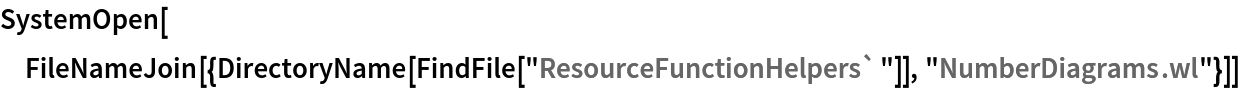Function Repository Resource:

# FractionPieChart

Visualize fractions and mixed numbers using pie charts

Contributed by: Wolfram|Alpha Math Team
 ResourceFunction["FractionPieChart"][n/d] creates a pie chart or row of pie charts representing the fraction n/d. ResourceFunction["FractionPieChart"][n] creates a pie chart or row of pie charts representing the integer n. ResourceFunction["FractionPieChart"][n1/d1 + n2/d2 + …] creates a row of pie charts representing the addition of the fractions n1/d1,n2/d2,….

## Details and Options

ResourceFunction["FractionPieChart"] accepts inputs greater than 1/50 and less than 50.
ResourceFunction["FractionPieChart"] has the same options as Graphics, with the following additions:
 FillingStyle sets the color for the filled parts of the pie charts "PieColor" sets the color for the unfilled parts of the pie charts "ShowAddition" Automatic determines whether to show plus signs between pie charts FontColor sets the color of the plus signs between pie charts “PlusFontSize" Automatic sets the size of the plus signs between pie charts "UnitLabels" Automatic sets the text of the label displayed in the sections of the pie charts
ResourceFunction["FractionPieChart"] has the attributes HoldAll and Listable.

## Examples

### Basic Examples (3)

Get a pie chart representing the fraction 4/5:

 In:=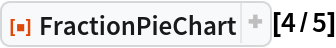Out=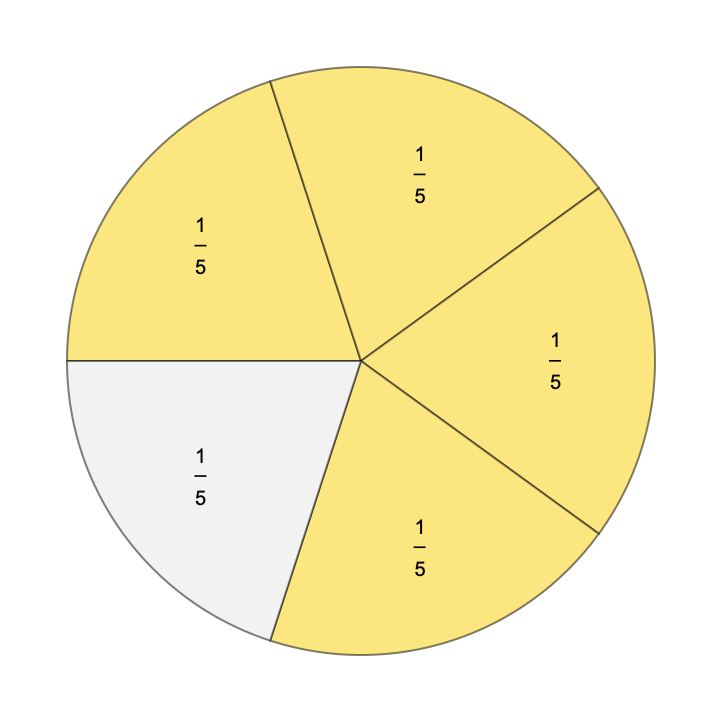Get a row of pie charts representing the fraction 8/3:

 In:=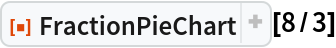Out=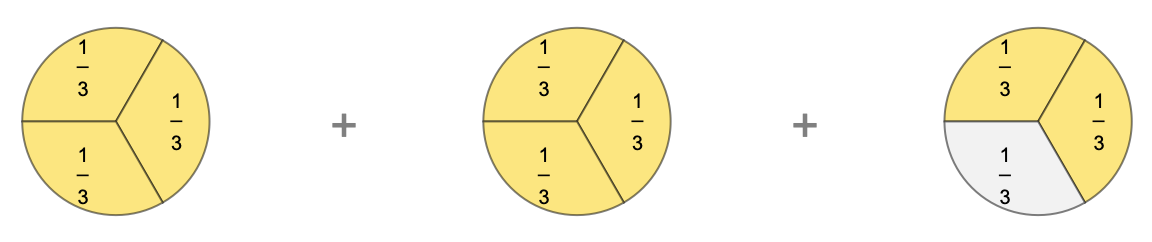Get a row of pie charts representing the addition 1/3 + 3/4 + 5/30:

 In:=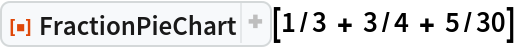Out=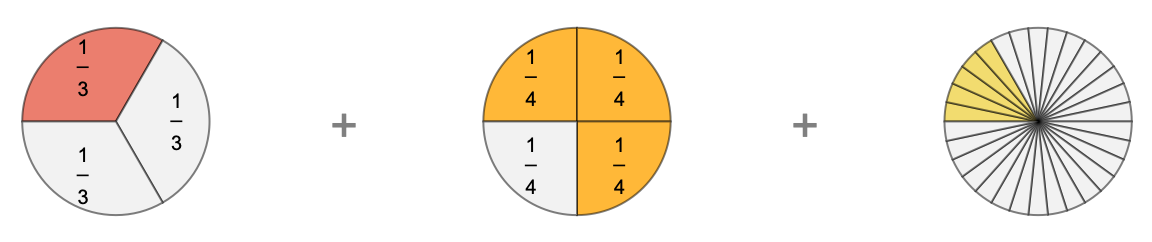### Scope (1)

Use FractionPieChart to visually compare the mixed number and improper fraction forms of a number:

 In:=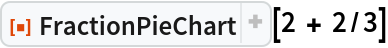Out=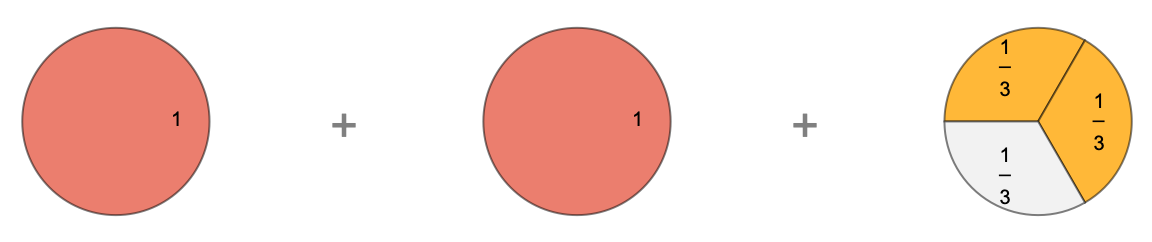In:=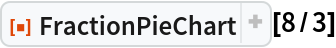Out=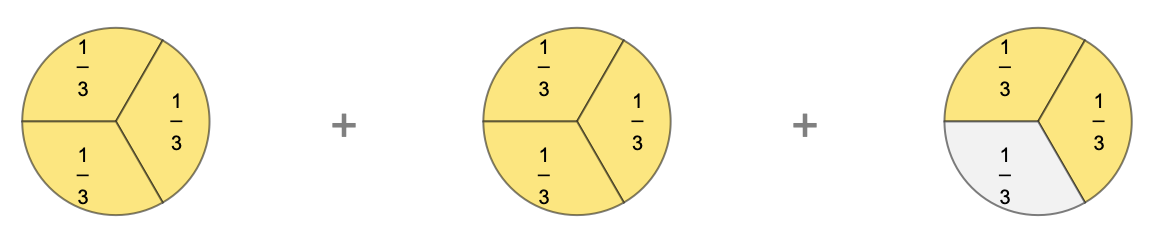### Options (11)

#### FillingStyle (2)

Use the FillingStyle option to set the color of the filled sections of the pie charts:

 In:=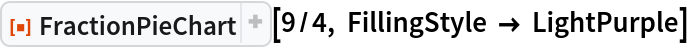Out=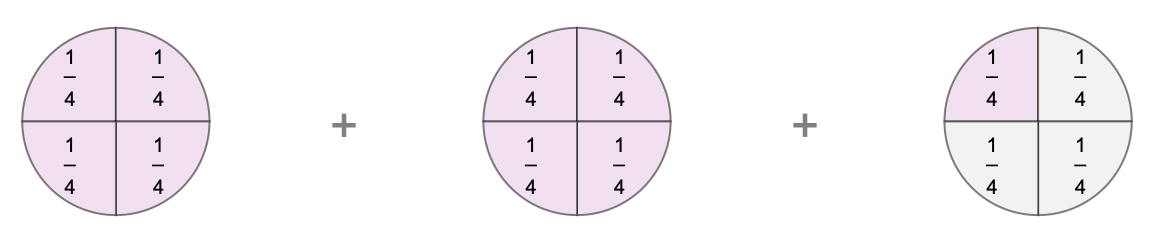For addition of multiple fractions, FillingStyle can be a list of colors:

 In:=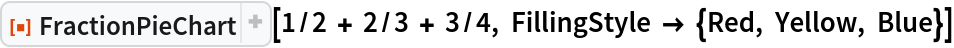Out=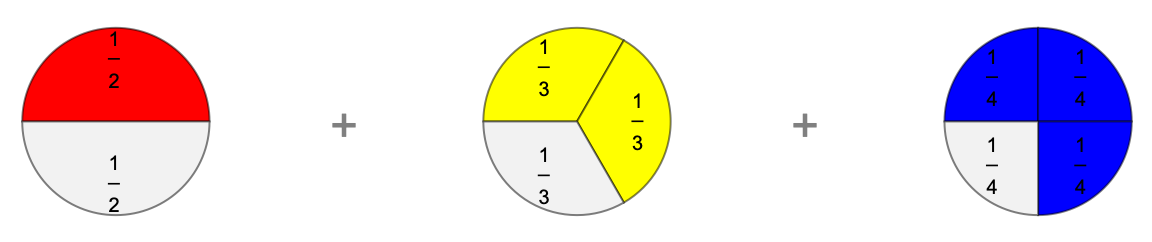#### PieColor (1)

Use the "PieColor" option to set the color of the unfilled sections of the pie charts:

 In:=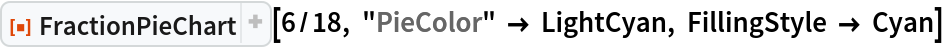Out=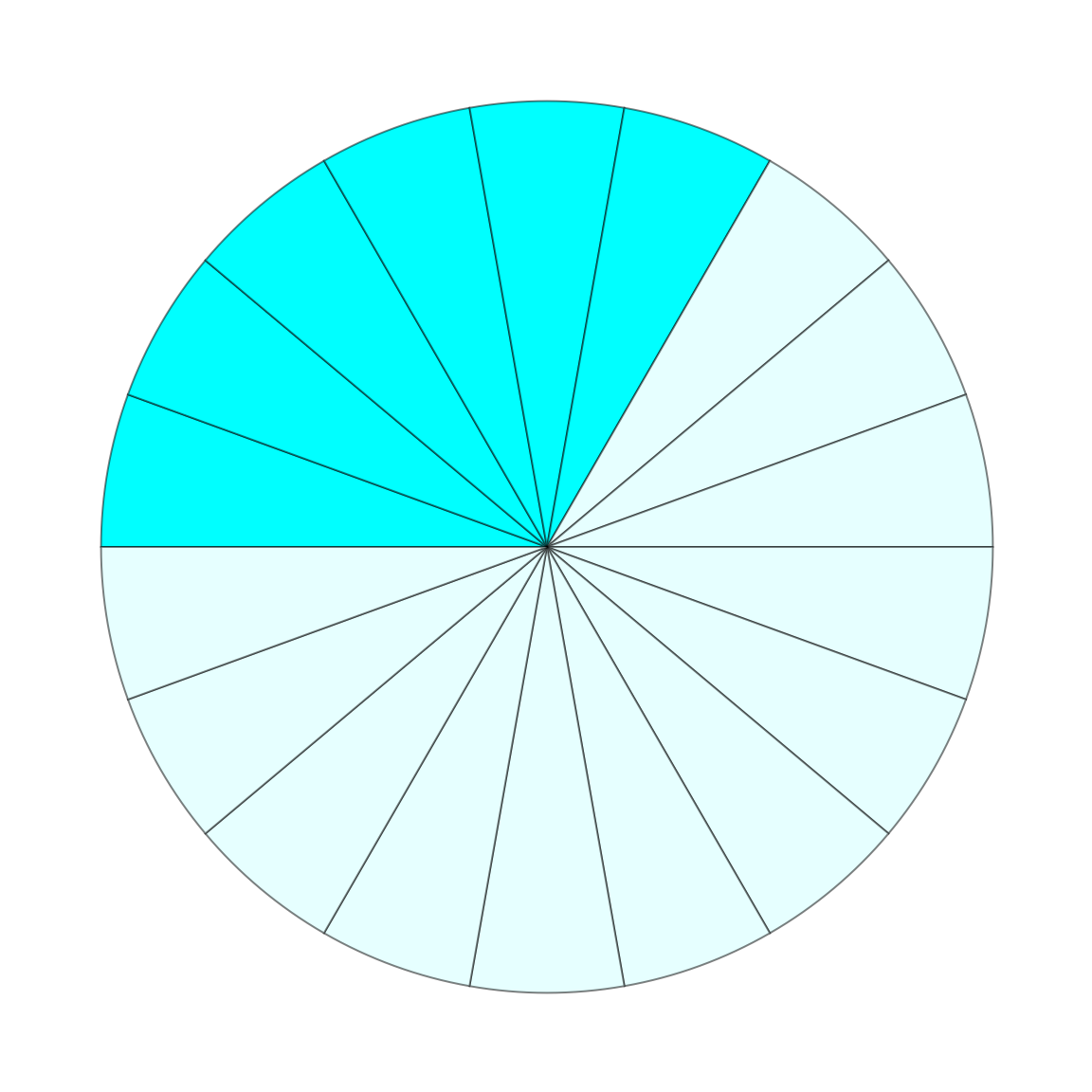Set the "ShowAddition" to False to hide the plus signs between multiple pie charts:

 In:=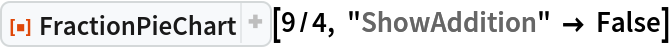Out=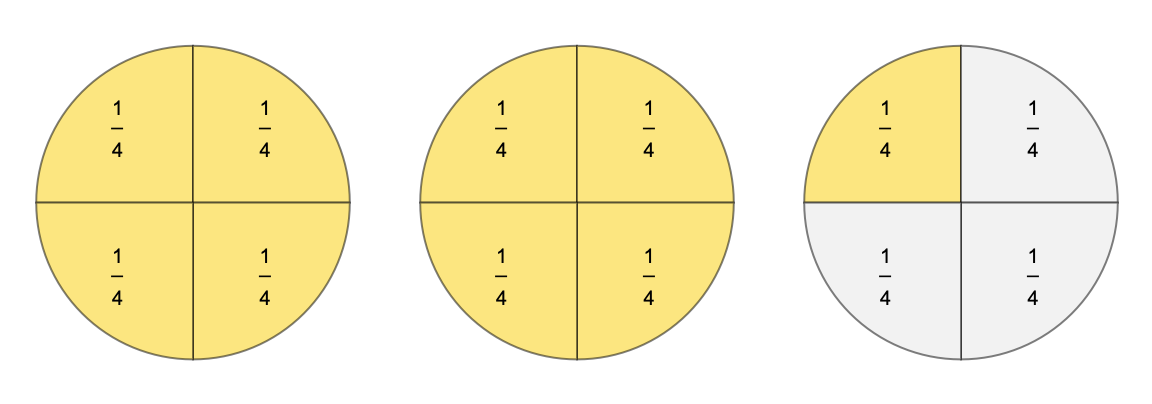For large numbers of pie charts, the plus signs are automatically hidden. Set the "ShowAddition" option to True to force addition symbols:

 In:=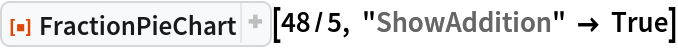Out=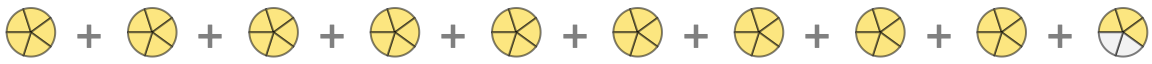#### FontColor (2)

Use the FontColor option to set the color of the plus signs between pie charts:

 In:=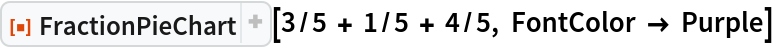Out=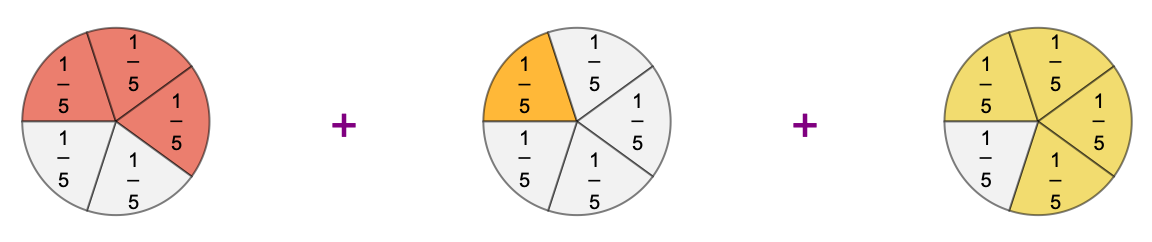To change the color of the labels font, use the LabelStyle option:

 In:=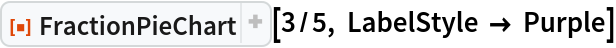Out=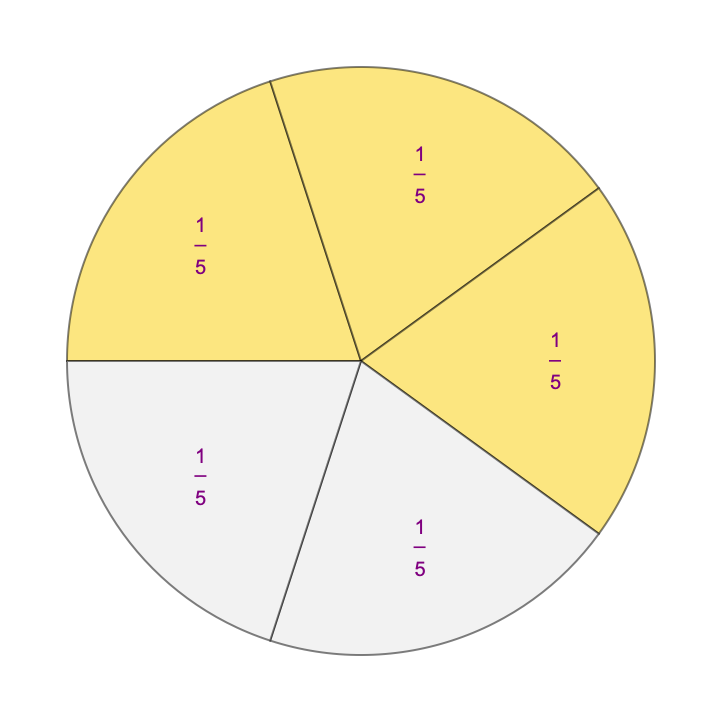#### PlusFontSize (1)

Use the "PlusFontSize" option to set the size of the plus signs between pie charts:

 In:=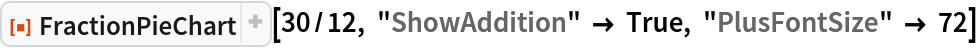Out=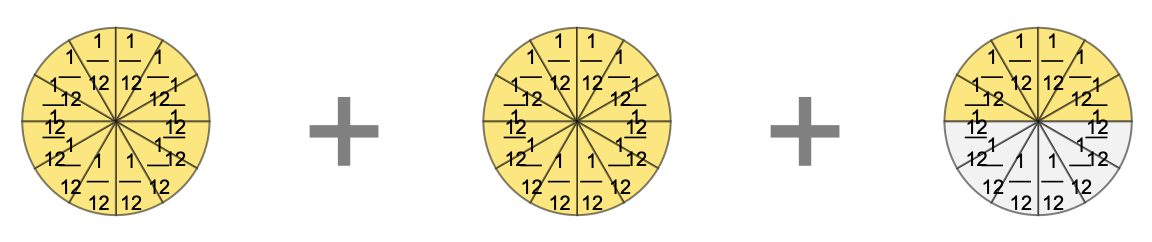#### UnitLabels (3)

Unit labels are automatically hidden for small sectors:

 In:=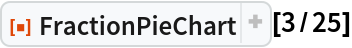Out=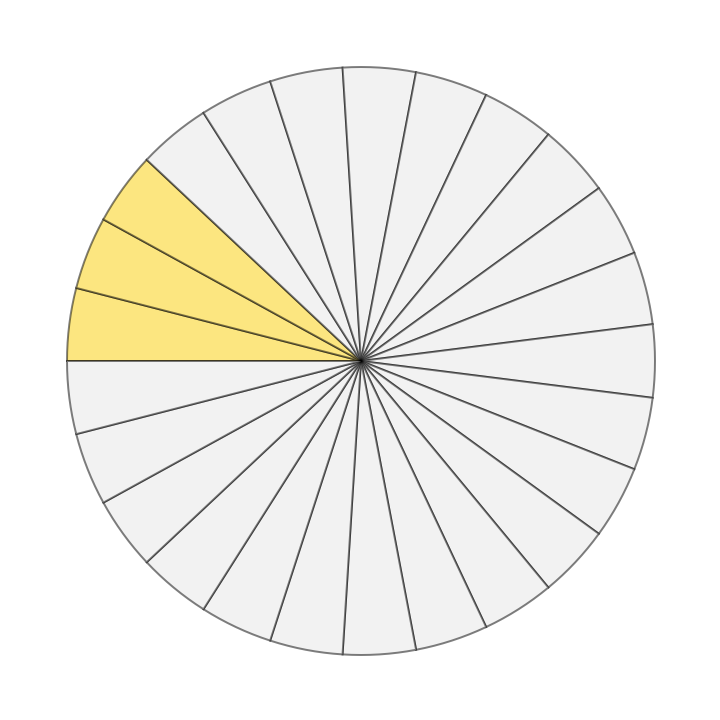Force showing unit labels by setting the "UnitLabels" option to True:

 In:=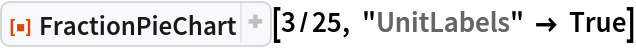Out=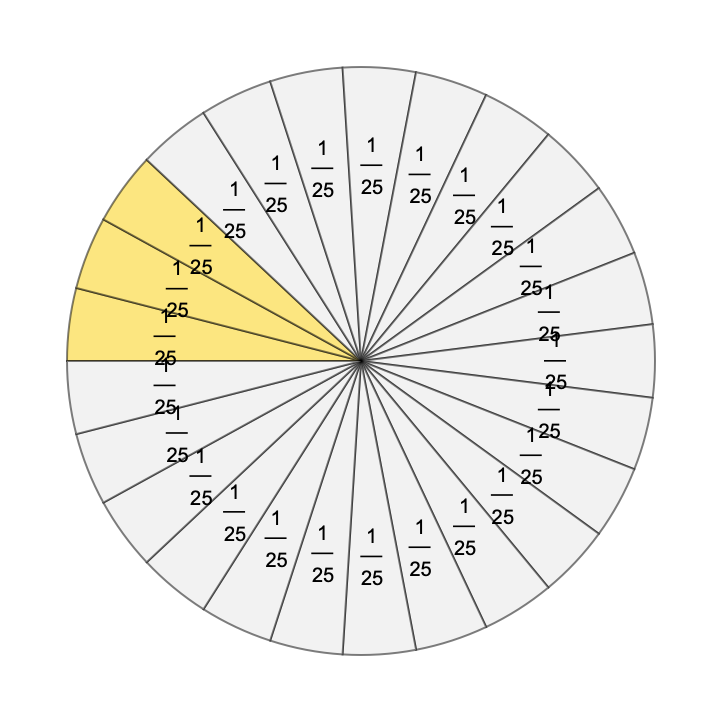Customize the unit labels via the "UnitLabels" option:

 In:=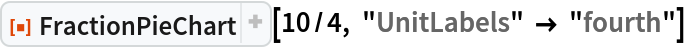Out=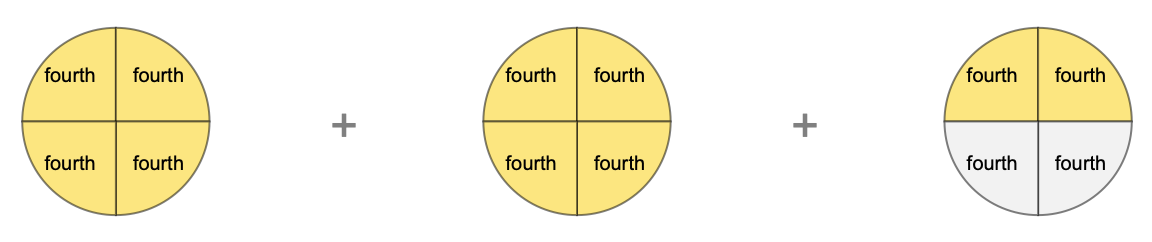## Publisher

Wolfram|Alpha Math Team

## Version History

• 2.0.0 – 23 March 2023
• 1.0.0 – 28 March 2022

## Author Notes

To view the full source code for FractionPieChart, evaluate the following:

 In:=